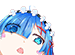# 换了主题 添加了下雪效果

in 冷轩纪 文章转载请注明来源！

``` (function() { function k(a, b, c) { if (a.addEventListener) a.addEventListener(b, c, false); else a.attachEvent && a.attachEvent("on" + b, c) } function g(a) { if (typeof window.onload != "function") window.onload = a; else { var b = window.onload; window.onload = function() { b(); a() } } } function h() { var a = {}; for (type in { Top: "", Left: "" }) { var b = type == "Top" ? "Y": "X"; if (typeof window["page" + b + "Offset"] != "undefined") a[type.toLowerCase()] = window["page" + b + "Offset"]; else { b = document.documentElement.clientHeight ? document.documentElement: document.body; a[type.toLowerCase()] = b["scroll" + type] } } return a } function l() { var a = document.body, b; if (window.innerHeight) b = window.innerHeight; else if (a.parentElement.clientHeight) b = a.parentElement.clientHeight; else if (a && a.clientHeight) b = a.clientHeight; return b } function i(a) { this.parent = document.body; this.createEl(this.parent, a); this.size = Math.random() * 5 + 5; this.el.style.width = Math.round(this.size) + "px"; this.el.style.height = Math.round(this.size) + "px"; this.maxLeft = document.body.offsetWidth - this.size; this.maxTop = document.body.offsetHeight - this.size; this.left = Math.random() * this.maxLeft; this.top = h().top + 1; this.angle = 1.4 + 0.2 * Math.random(); this.minAngle = 1.4; this.maxAngle = 1.6; this.angleDelta = 0.01 * Math.random(); this.speed = 2 + Math.random() } var j = false; g(function() { j = true }); var f = true; window.createSnow = function(a, b) { if (j) { var c = [], m = setInterval(function() { f && b > c.length && Math.random() < b * 0.0025 && c.push(new i(a)); ! f && !c.length && clearInterval(m); for (var e = h().top, n = l(), d = c.length - 1; d >= 0; d--) if (c[d]) if (c[d].top < e || c[d].top + c[d].size + 1 > e + n) { c[d].remove(); c[d] = null; c.splice(d, 1) } else { c[d].move(); c[d].draw() } }, 40); k(window, "scroll", function() { for (var e = c.length - 1; e >= 0; e--) c[e].draw() }) } else g(function() { createSnow(a, b) }) }; window.removeSnow = function() { f = false }; i.prototype = { createEl: function(a, b) { this.el = document.createElement("img"); this.el.setAttribute ("src", b + "http://mimg.127.net/hxm/quan/hd/111207_sdj/style/img/snow.gif"); this.el.style.position = "absolute"; this.el.style.display = "block"; this.el.style.zIndex = "99999"; this.parent.appendChild(this.el) }, move: function() { if (this.angle < this.minAngle || this.angle > this.maxAngle) this.angleDelta = -this.angleDelta; this.angle += this.angleDelta; this.left += this.speed * Math.cos(this.angle * Math.PI); this.top -= this.speed * Math.sin(this.angle * Math.PI); if (this.left < 0) this.left = this.maxLeft; else if (this.left > this.maxLeft) this.left = 0 }, draw: function() { this.el.style.top = Math.round(this.top) + "px"; this.el.style.left = Math.round(this.left) + "px" }, remove: function() { this.parent.removeChild(this.el); this.parent = this.el = null } } })(); createSnow("", 40); ```0:00